# ' )y" + 6xy = 0 about x。:0. #2.) (15 points) For ( 1-X Find two linearly independent solutions y,(x) and V2(x)...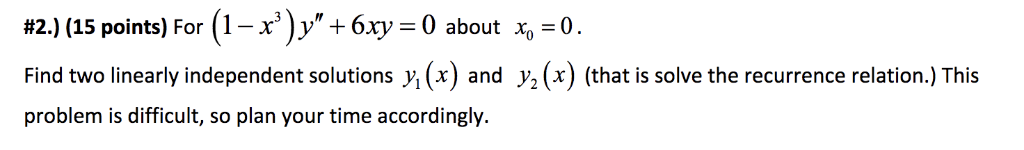' )y" + 6xy = 0 about x。:0. #2.) (15 points) For ( 1-X Find two linearly independent solutions y,(x) and V2(x) (that is solve the recurrence relation.) This problem is difficult, so plan your time accordingly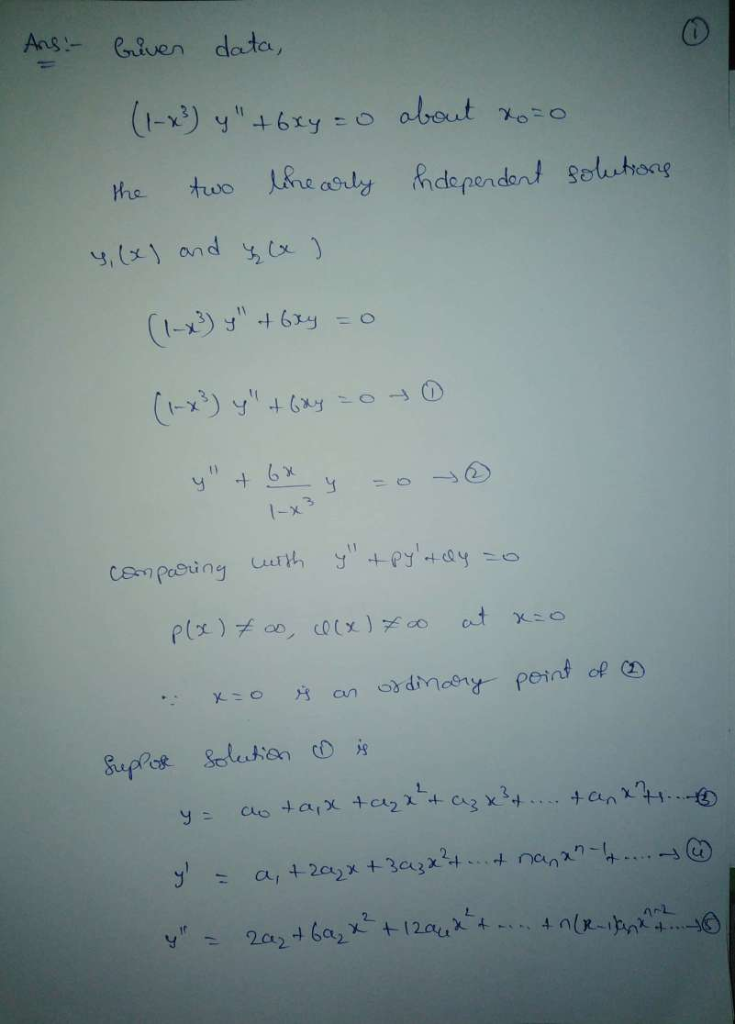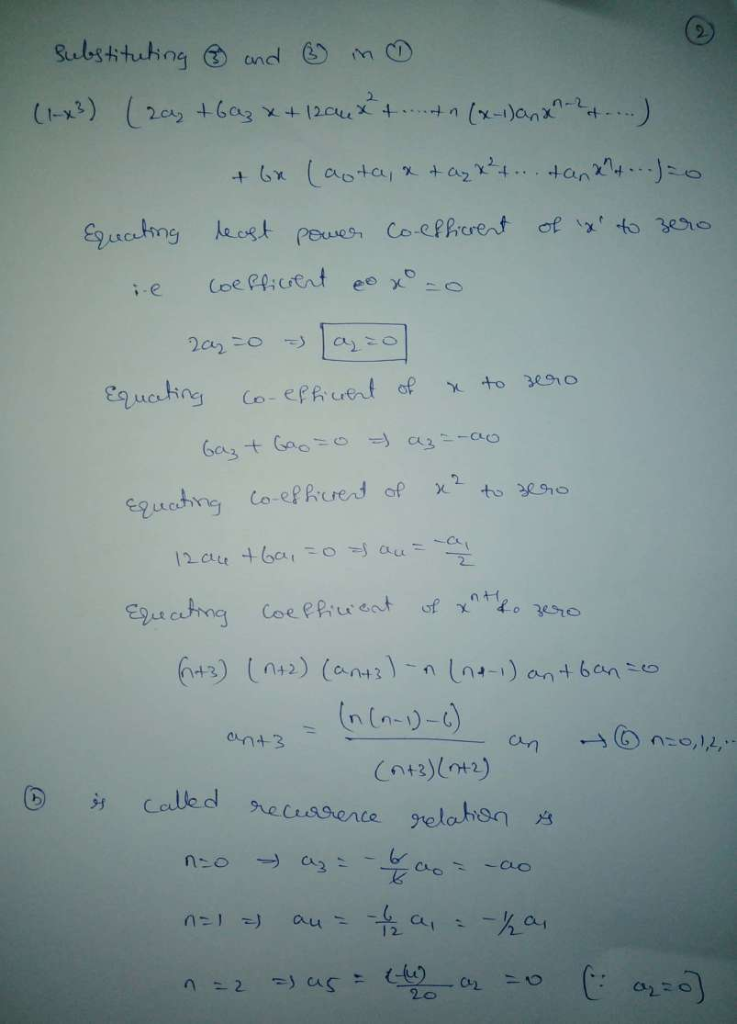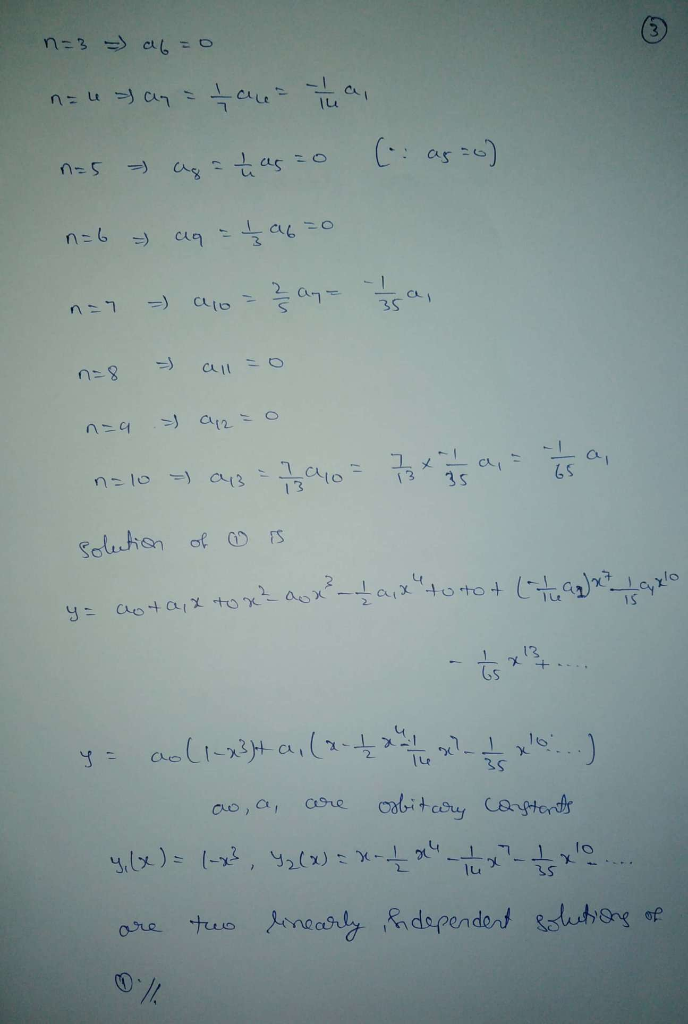##### Add Answer of: ' )y" + 6xy = 0 about x。:0. #2.) (15 points) For ( 1-X Find two linearly independent solutions y,(x) and V2(x)...
Similar Homework Help Questions
• ### Given the DE: y"-(x+1)y'-y=0 use it to answer the following: a) Find the singular point(s), if...

Given the DE: y"-(x+1)y'-y=0 use it to answer the following: a) Find the singular point(s), if any, and if lower bound for the radius of convergence for a power series solution about the ordinary points x=0 b)The recurrence relation Hint: It will be a 3-term recurrence relation c)Give the first four non-zero terms of each of the two linearly independent power series solutions near the ordinary point x=0

• ### (6 points) Use the method of Frobenius to obtain linearly independent series solutions about x = 0 use an initial index of k = 1 to develop the recurrence relations The indicial roots are(in ascendin...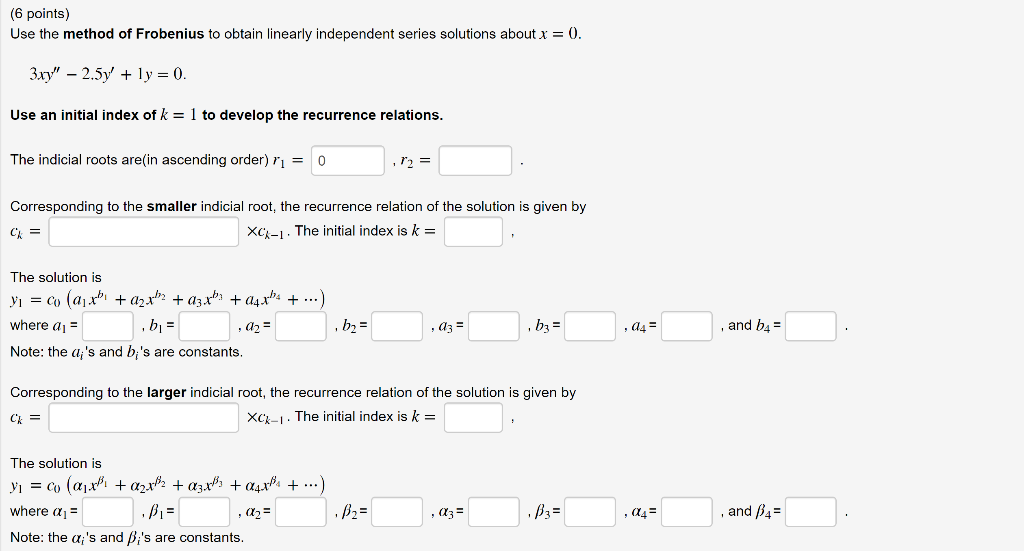(6 points) Use the method of Frobenius to obtain linearly independent series solutions about x = 0 use an initial index of k = 1 to develop the recurrence relations The indicial roots are(in ascending order) rl = 0 Corresponding to the smaller indicial root, the recurrence relation of the solution is given by xck-1-The initial index is k The solution is , and b4 b3 where ai Note: the a's and b 's are constants Corresponding to the larger...

• ### Find two power series solutions of the given differential equation about the ordinary point x=0. (x^2+2)y"+6xy'-y=0...

Find two power series solutions of the given differential equation about the ordinary point x=0. (x^2+2)y"+6xy'-y=0 (Show all steps using y= please) nfiniti

• ### I. Let 2r2y"-ry' + (r' + 1) y = 0. ) Verify that a 0 is a regular singular point. (2 points) ) Find two linearly independent power series solutions about a0. Provide at least3 aon-ze...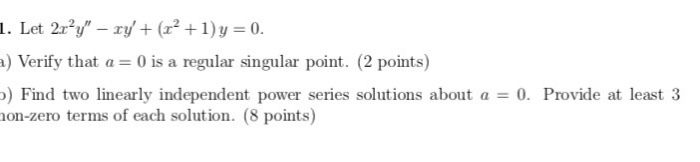I. Let 2r2y"-ry' + (r' + 1) y = 0. ) Verify that a 0 is a regular singular point. (2 points) ) Find two linearly independent power series solutions about a0. Provide at least3 aon-zero terms of each solution. (8 points) 0 0 is reowlar 터 -2 kti I'm Stuck-HEKG-HER- ME FINISH I. Let 2r2y"-ry' + (r' + 1) y = 0. ) Verify that a 0 is a regular singular point. (2 points) ) Find two linearly independent...

• ### Solve y" _ 2ry'-2y 0 by me each of the two linearly independent solutions unless the series termi...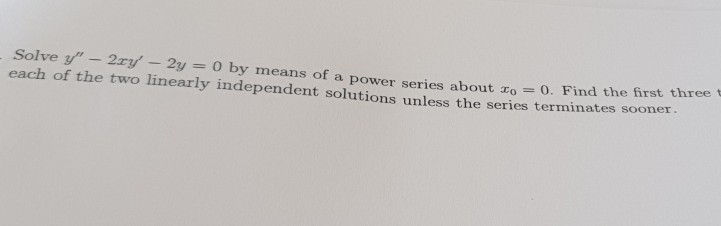need help with Calculus assignment Solve y" _ 2ry'-2y 0 by me each of the two linearly independent solutions unless the series terminates sooner ans of a power series about zo 0, Find the first three t Solve y" _ 2ry'-2y 0 by me each of the two linearly independent solutions unless the series terminates sooner ans of a power series about zo 0, Find the first three t

• ### Find two linearly independent solutions to the differential equation

the differential equation is(4-x^2)y'' -xy' -4y =0Find two linearly independent solutions where:1. y1(0) =1 and y1'(0)=0and2. y2(0)=0 and y2'(0)=1

• ### Find two linearly independent Frobenius series solutions if possible

Show there is a regular singular point at x0+0 and find two linearly independent Frobenius series solutions if two exist.x^2y'' +xy' + (x^4 - 4)y +0

• ### 4. Verify that yi xPJp(x) and y2 - xPYp(x) are linearly independent solutions of xy" + (1-2 )y, +...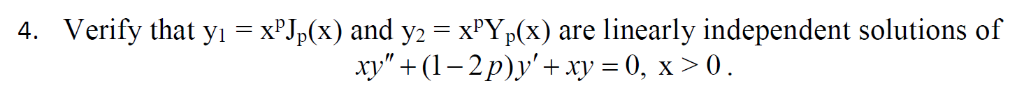4. Verify that yi xPJp(x) and y2 - xPYp(x) are linearly independent solutions of xy" + (1-2 )y, + xy-0, x > 0. 4. Verify that yi xPJp(x) and y2 - xPYp(x) are linearly independent solutions of xy" + (1-2 )y, + xy-0, x > 0.

• ### Verify that yi = XpJp(x) and y, = xryp(x) are linearly independent solutions of xy" + (1-2p)y, + ...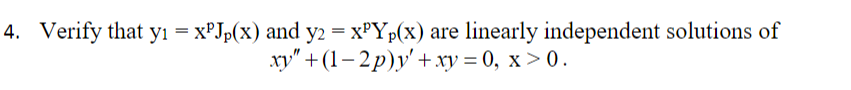Verify that yi = XpJp(x) and y, = xryp(x) are linearly independent solutions of xy" + (1-2p)y, + xy = 0, x>0. 4. Verify that yi = XpJp(x) and y, = xryp(x) are linearly independent solutions of xy" + (1-2p)y, + xy = 0, x>0. 4.

• ### find 2 linearly independent solutions to the given differential equation

FIND TWO LINEARLY INDEPENDENT SOLUTIONS TO THE GIVENDIFFERENTIAL EQUATIONxy'' + y' - y = 0

Need Online Homework Help?